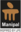Trending ▼   ResFinder# ICSE Class X Prelims 2020 : Mathematics (Madhusthali Vidyapeeth, Deoghar)

1 pages, 13 questions, 0 questions with responses, 0 total responses,00Soumya Roy Sikkim Manipal University (SMU), Gangtok Master of Computer Application Computer Application
+Fave Message
 Home > coolsroy_mdp >   F Also featured on: School PageFormatting page ...

VIBRANT PREP CLASSES(MATHS-10 TEST) 1. The 4th term of a G.P. is 16 and the 7th term is 128. Find the first term and common ratio of the series. 2. A man invests Rs. 22,500 in Rs. 50 shares available at 10% discount. If the dividend paid by the company is 12%, calculate:  (i) The number of shares purchased (ii) The annual dividend received. (iii) The rate of return he gets on his investment. Give your answer correct to the nearest whole number. 3. On a map drawn to a scale of 1 : 50,000, a rectangular plot of land ABCD has the following dimensions. AB = 6 cm; BC = 8 cm and all angles are right angles. Find:  (i) the actual length of the diagonal distance AC of the plot in km. (ii) the actual area of the plot in sq. km. 4. A(2, 5), B( 1, 2) and C(5, 8) are the vertices of a triangle ABC, `M' is a point on AB such that AM : MB = 1 : 2. Find the co-ordinates of 'M . Hence find the equation of the line passing through the points C and M.  5. If the mean of the following distribution is 24, find the value of 'a '. 6. Using ruler and compass only, construct a ABC such that BC = 5 cm and AB = 6.5 cm and ABC = 120  (i) Construct a circum-circle of ABC (ii) Construct a cyclic quadrilateral ABCD, such that D is equidistant from AB and BC. 7. In PQR, MN is parrallel to QR and (ii) Prove that OMN and ORQ are similar. (iii) Find, Area of OMN : Area of ORQ 8. The angle of elevation from a point P of the top of a tower QR, 50 m high is 60o and that of the tower PT from a point Q is 30 . Find the height of the tower PT, correct to the nearest metre.  9. The mean of following number is 68. Find the value of x . 45, 52, 60, x, 69,70,26,81 and 94. Hence estimate the median. 10. In the given figure PQRS is a cyclic quadrilateral PQ and SR produced meet at T.  (i) Prove TPS TRQ. (ii) Find SP if TP = 18 cm, RQ = 4 cm and TR = 6 cm. (iii) Find area of quadrilateral PQRS if area of PTS = 27 cm2.